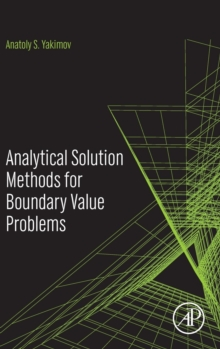Supporting your high street Find out how »
• My Account# Analytical Solution Methods for Boundary Value Problems Hardback

#### Description

Analytical Solution Methods for Boundary Value Problems is an extensively revised, new English language edition of the original 2011 Russian language work, which provides deep analysis methods and exact solutions for mathematical physicists seeking to model germane linear and nonlinear boundary problems.

Current analytical solutions of equations within mathematical physics fail completely to meet boundary conditions of the second and third kind, and are wholly obtained by the defunct theory of series.

These solutions are also obtained for linear partial differential equations of the second order.

They do not apply to solutions of partial differential equations of the first order and they are incapable of solving nonlinear boundary value problems. Analytical Solution Methods for Boundary Value Problems attempts to resolve this issue, using quasi-linearization methods, operational calculus and spatial variable splitting to identify the exact and approximate analytical solutions of three-dimensional non-linear partial differential equations of the first and second order.

The work does so uniquely using all analytical formulas for solving equations of mathematical physics without using the theory of series.

Within this work, pertinent solutions of linear and nonlinear boundary problems are stated.

On the basis of quasi-linearization, operational calculation and splitting on spatial variables, the exact and approached analytical solutions of the equations are obtained in private derivatives of the first and second order.

Conditions of unequivocal resolvability of a nonlinear boundary problem are found and the estimation of speed of convergence of iterative process is given.

On an example of trial functions results of comparison of the analytical solution are given which have been obtained on suggested mathematical technology, with the exact solution of boundary problems and with the numerical solutions on well-known methods.

#### Information

• Format: Hardback
• Pages: 200 pages
• Publisher: Elsevier Science Publishing Co Inc
• Publication Date:
• Category: Differential calculus & equations
• ISBN: 9780128042892

£68.99

£56.09

on all orders

###### Pick up orders

from local bookshops